Click here to register for the 2022 GLOBAL MATHS AND SCIENCE LESSON ON TUESDAY 11 OCTOBER. See below for links to lesson resources in English and Spanish.

Sheep Talk  The first sheep talk word is A.To find the next term in the word sequence, each A is replaced by B and each B is replaced by AB. The rule is:   A -> B   and    B-> AB

### Here are the first 7 words in the sheep talk sequence: A    B    AB    BAB    ABBAB    BABABBAB    ABBABBABABBAB

Count the number of occurrences of A, of B, and the total number of letters, and fill in the table. Can you spot the pattern in the sequences?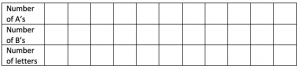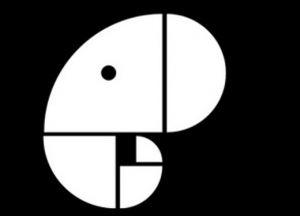Elephant Dreaming Copy the picture below on squared paper, draw the spiral and create your own elephant.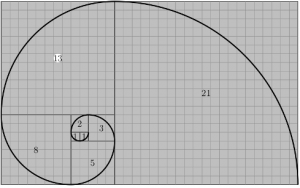The numbers in the centres of the squares give the radii of the quarter circles you need to draw

For the elephant’s trunk, start from the quarter circle of radius 3 and don’t draw the quarter circles with radii 1, 1, and 2.

ESPAÑOL – hacer clic aquí para bajar la lección global de 30 minutos

The Fibonacci Sequence 1, 1, 2, 3, 5, 8, 13,…

Continue the sequence and work out the ratio of each term to the term before it. What do you notice? Use a spreadsheet to do the calculations.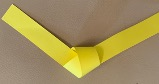### If you draw a pentagon accurately can you find ratios of lengths equal to the Golden Ratio?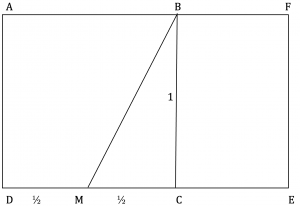Draw a Golden Rectangle

1. Draw a square ABCD and take the length of the edges of the square as 1 unit.
2. Extend the line DC.
3. Mark M the midpoint of DC.
4. Join MB.
5. Draw an arc centre M radius MB cutting the extended line DC at point E.
6. Mark E on DC so ME=MB

1. Calculate length MB
2. Calculate lengths DE and CE
3. Calculate the golden ratio DE/AD in surd form and to 3 decimal places.

Calculate the ratio BC/CE in surd form and to 3 decimal places.

What do you notice?

The two ratios DE/AD and BC/CE should be the same, the Golden Ratio.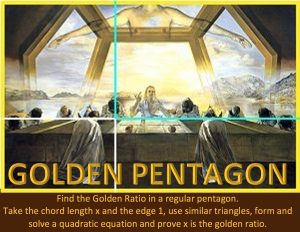The Last Supper by Salvador Dali (1955).

Notice the proportions of the rectangles in this painting. Also notice the pentagon. It is actually part of a dodecahedron. The Golden Ratio occurs in many ratios in a pentagon.

Do some research on the internet about the Golden Ratio in art.

Tagged with: